# 水翼尾缘摆动建模与流体动力特性研究Modeling and Hydrodynamic Characteristics of Flapping Hydrofoil Trailing Edge

DOI: 10.12677/MOS.2021.101016, PDF, HTML, XML, 下载: 39  浏览: 357  科研立项经费支持

Abstract: The hydrofoil in the swinging state is widely used in the fields of propeller, wave energy capture and bionic propulsion, and its hydrodynamic characteristic is one of the factors that must be considered in the design of the device. In this paper, the hydrodynamic characteristics of the flapping hydrofoil under different swing angles and different incoming flow speeds are simulated and analyzed. First, the NACA0012 airfoil is established as the analysis object, and then the hydrodynamic calculation method of the flapping hydrofoil is obtained by using the polyhedral grid and the dynamic grid technology. On this basis, the hydrodynamic characteristics of the hydrofoil in the swing angle range of ±20˚ and the flow velocity range of 1~10 m/s are simulated and analyzed. The results show that as the swing angle increases, the maximum pressure changes less, the minimum pressure gradually increases, both lift and resistance increase, the speed of lift increase gradually decreases, and the speed of resistance increase gradually increases. With the increase of the incoming flow velocity, the maximum pressure and the minimum pressure (negative pressure) both increase to varying degrees, the lift and resistance increase, and the increasing speed gradually increases.

1. 引言

2. 几何模型

2.1. 水翼几何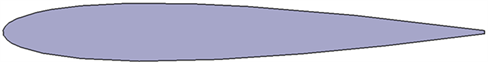Figure 1. Hydrofoil geometry

2.2. 计算工况

3. 计算模型

3.1. 控制方程

$\text{\hspace{0.17em}}\frac{\partial \rho }{\partial t}+\nabla \cdot \left(\rho \stackrel{\to }{V}\right)=0$ (1)

$\frac{\partial \left(\rho u\right)}{\partial t}+\nabla \cdot \left(\rho u\stackrel{\to }{V}\right)=\frac{\partial p}{\partial x}+\frac{\partial {\tau }_{xx}}{\partial x}+\frac{\partial {\tau }_{yx}}{\partial y}+\frac{\partial {\tau }_{zx}}{\partial z}+\rho {f}_{x}$ (2)

$\frac{\partial \left(\rho v\right)}{\partial t}+\nabla \cdot \left(\rho v\stackrel{\to }{V}\right)=-\frac{\partial p}{\partial y}+\frac{\partial {\tau }_{xy}}{\partial x}+\frac{\partial {\tau }_{yy}}{\partial y}+\frac{\partial {\tau }_{zy}}{\partial z}+\rho {f}_{y}$ (3)

$\frac{\partial \left(\rho w\right)}{\partial t}+\nabla \cdot \left(\rho w\stackrel{\to }{V}\right)=-\frac{\partial p}{\partial z}+\frac{\partial {\tau }_{xz}}{\partial x}+\frac{\partial {\tau }_{yz}}{\partial y}+\frac{\partial {\tau }_{zz}}{\partial z}+\rho {f}_{z}$ (4)

$\frac{\partial }{\partial t}\left(\rho k\right)+\frac{\partial }{\partial {x}_{i}}\left(\rho k{u}_{i}\right)=\frac{\partial }{\partial {x}_{j}}\left({\alpha }_{k}{\mu }_{eff}\frac{\partial k}{\partial {x}_{j}}\right)+{G}_{k}+{G}_{b}-\rho e-{Y}_{M}+{S}_{k}$ (5)

$\frac{\partial }{\partial t}\left(\rho e\right)+\frac{\partial }{\partial {x}_{i}}\left(\rho e{u}_{i}\right)=\frac{\partial }{\partial {x}_{j}}\left({\alpha }_{e}{\mu }_{eff}\frac{\partial e}{\partial {x}_{j}}\right)+{C}_{1e}\frac{e}{k}\left({G}_{k}+{C}_{3e}{G}_{b}\right)-{C}_{2e}\rho \frac{{e}^{2}}{k}-{R}_{e}+{S}_{e}$ (6)

Gk是由层流速度梯度而产生的湍流动能，Gb是由浮力而产生的湍流动能，YM由于在可压缩湍流中，过渡的扩散产生的波动，C1，C2，C3，为常量，αk和αe是k方程和ε方程的湍流Prandtl数。

3.2. 计算网格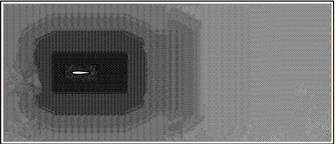(a)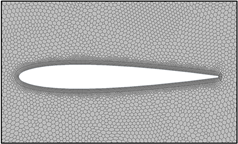(b)

Figure 2. Mesh: (a) Mesh of computational domain; (b) Mesh of boundary layer

4. 计算结果与分析

4.1. 不同水翼摆角流场特性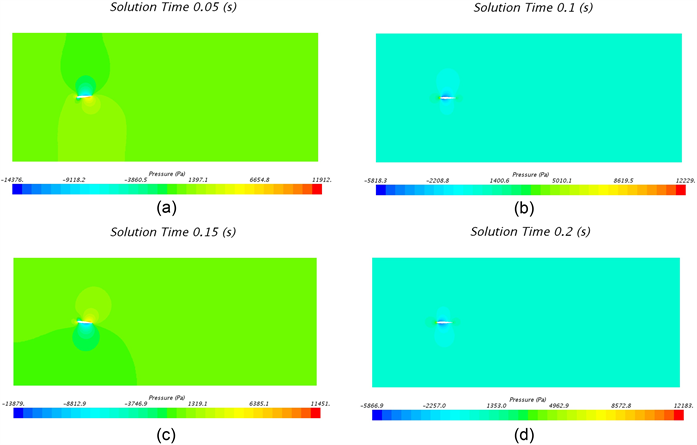Figure 3. Pressure contour of hydrofoil at 5˚ swing: (a) 0.05 s; (b) 0.1 s; (c) 0.15 s; (d) 0.2 s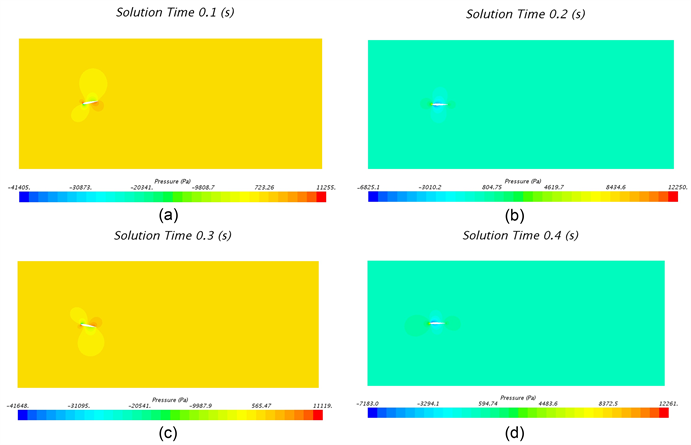Figure 4. Pressure contour of hydrofoil at 10˚ swing: (a) 0.1 s; (b) 0.2 s; (c) 0.3 s; (d) 0.4 s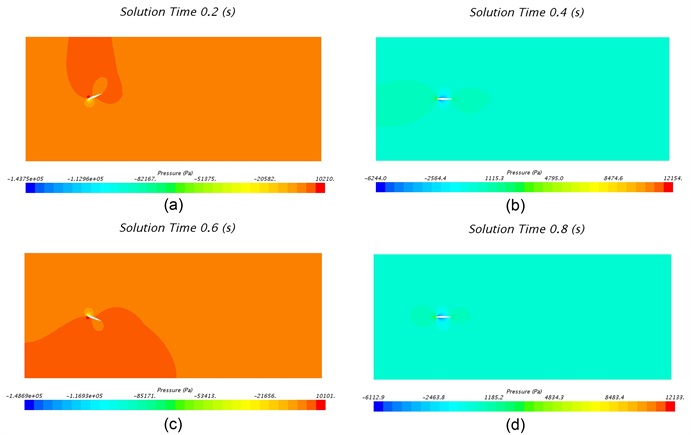Figure 5. Pressure contour of hydrofoil at 20˚ swing: (a) 0.2 s; (b) 0.4 s; (c) 0.6 s; (d) 0.8 s

4.2. 不同进流流场特性Figure 6. Pressure contour at 1 m/s inlet flow: (a) 0.1 s; (b) 0.2 s; (c) 0.3 s; (d) 0.4 sFigure 7. Pressure contour at 10 m/s inlet flow: (a) 0.1 s; (b) 0.2 s; (c) 0.3 s; (d) 0.4 s

4.3. 水翼受力结果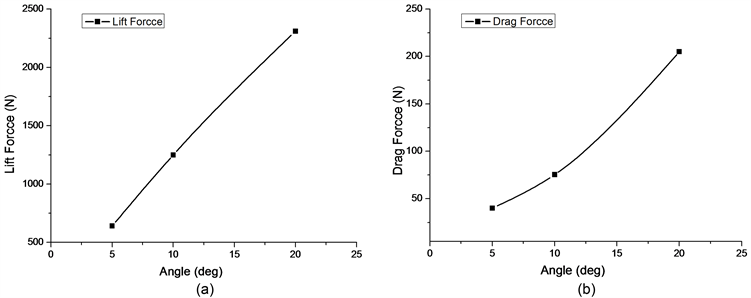Figure 8. Lift force and drag force of the hydrofoil at different swing angle: (a) Lift force; (b) Drag force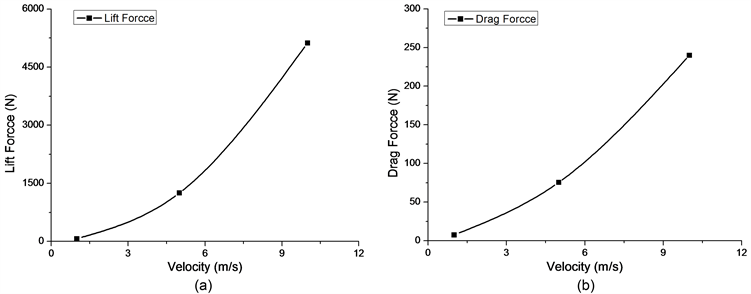Figure 9. Lift force and drag force of the hydrofoil at different flow velocity: (a) Lift force; (b) Drag force

5. 结论

1) 在不同摆动角度工况下，压力分布和速度分布相互对应，流速较大的区域为低压区域，随着摆动角度的增大，最大压力变化较小，最低压力(负压)逐渐增大。

2) 在不同来流速度工况下，随着来流速度的增大，最大压力和最小压力(负压)均有不同程度的增大。

3) 通过对不同工况的升力和阻力曲线分析，随着摆动角度在5˚~20˚范围内增大，升力和阻力均增大，升力增加的速度逐渐减小，阻力增加的速度逐渐增大；随着来流速度在1~10 m/s范围增大，升力和阻力增大，且增加的速度逐渐增大。

NOTES

*通讯作者。

  Anderson, J., Streitlien, K., Barrett, D. and Triantafyllou, M. (1998) Oscillating Foils of High Propulsive Efficiency. Journal of Fluid Mechanics, 360, 41-72. https://doi.org/10.1017/S0022112097008392  Young, J. and Lai, J.C.S. (2004) Oscillation Frequency and Amplitude Effects on the Wake of a Plunging Airfoil. AIAA Journal, 42, 2042-2052. https://doi.org/10.2514/1.5070  Ashraf, M.A., Young, J. and Lai, J.C.S. (2009) Effect of Airfoil Thickness, Camber and Reynolds Number on Plunging Airfoil Propulsion. 47th AIAA Aerospace Sciences Meeting In-cluding the New Horizons Forum and Aerospace Exposition, Orlando, Florida, 5-8 January 2009. https://doi.org/10.2514/6.2009-1274  Xiao, Q. and Liao, W. (2010) Numerical Investigation of Angle of Attack Profile on Propulsion Performance of an Oscillating Foil. Computers & Fluids, 39, 1366-1380. https://doi.org/10.1016/j.compfluid.2010.04.006  刘志恒. 二维水翼的水动力性能研究及扑翼试验装置设计[D]: [博士学位论文]. 武汉: 华中科技大学, 2007.  刘焕兴, 苏玉民, 庞永杰. 非正弦摆动对水翼水动力性能的影响[J]. 华中科技大学学报(自然科学版), 2016, 44(2): 15-20.  马鹏磊. 振荡翼的水动力特性及捕能机理研究[D]: [博士学位论文]. 济南: 山东大学, 2019.  胡健, 赵旺, 王子斌. 三维摆动水翼仿生推进水动力分析[J]. 应用科技, 2019, 46(2): 1-6.  张国政. 二维刚性翼摆动推进的水动力分析与实验研究[D]: [博士学位论文]. 哈尔滨: 哈尔滨工业大学, 2018.  郭春雨, 张佐天, 徐佩, 曹绪祥. 改进型振荡水翼水动力试验及机理[J]. 华中科技大学学报(自然科学版), 2019, 47(4): 87-93.